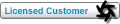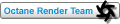## [ScriptGraph] GetInputValue from an input node Tweet

Forum for OctaneRender Lua scripting examples, discussion and support.

### [ScriptGraph] GetInputValue from an input node

Hello,

Sorry for this noob question. I tried to find as much information as I can about how to do this. I though I understood it but I keep getting errors, so I'm requesting your help.

What I'm trying to achieve:
Using a Transform Scale node to randomize some transformation I'm doing.
It was working well using a basic float input like I'm doing for the translation and rotation. But I wanted the possibility to lock the 3 values together like we have with a Transform scale.

However, I'm having trouble accessing the float values of the Transform Scale node

Code: Select all
`function ScriptGraph.onInit(self, graph)    local inputs = self:setInputLinkers(        {            {                label           = "Random Translation",                type            = octane.PT_FLOAT,                defaultNodeType = octane.NT_FLOAT,                defaultValue    = { 0, 0, 0 },                sliderBounds    = { 0, 1 },                logarithmic     = true,            },            {                label           = "Random Rotation",                type            = octane.PT_FLOAT,                defaultNodeType = octane.NT_FLOAT,                defaultValue    = { 0, 0, 0 },                sliderBounds    = { 0, 1 },                logarithmic     = true,            },            {                label           = "Random Scale",                type            = octane.PT_TRANSFORM,                defaultNodeType = octane.NT_TRANSFORM_SCALE,                defaultValue    = { 0, 0, 0 },                sliderBounds    = { 0, 10 },                logarithmic     = true,            },            {                label           = "Seed",                type            = octane.PT_INT,                defaultNodeType = octane.NT_INT,                defaultValue    = { 1 },                sliderBounds    = { 1,1000 },                logarithmic     = true,            },        })    IN_TRANSLATION_RAND = inputs    IN_ROTATION_RAND = inputs    IN_SCALE_RAND = inputs    IN_SEED = inputs    local randTrans = self:getInputValue(IN_TRANSLATION_RAND)    local randRot = self:getInputValue(IN_ROTATION_RAND)    local randScale = self:getConnectedNode(IN_SCALE_RAND):getInputValue(octane.P_SCALE)`

I'm not pasting the entire code but just the areas that concern my issue.
It was perfectly working until I tried to improve a bit my script.

At this line:
Code: Select all
`    local randScale = self:getConnectedNode(IN_SCALE_RAND):getInputValue(octane.P_SCALE)`

What I'm trying to store in this variable is an array of value like I can get with my randTrans and randRot variables.
I tried a lot of different syntax and combination without success.

The error on this one is

Code: Select all
`attempt to call a nil value (method 'getConnectedNode')`

Thank you very much for your help!
RenartPosts: 70
Joined: Mon Jul 04, 2016 8:21 pm

### Re: [ScriptGraph] GetInputValue from an input node

Call `graph:getConnectedNode()`.

The types of the passed in parameters are:

• `self` → octane.scriptgraph (this is the table which is returned from the script embedded in the scripted graph)
• `graph` → octane.nodegraph (this is the node graph, so you can get input nodes, etc.)

You can open the API browser to get help for either type.

Your `IN_*` variables will contain the input linker nodes, these have one pin with id P_INPUT. So you can call `IN_SCALE_RAND:getInputNode(octane.P_INPUT)` to get the node connected to that input.

For the transforms, use `self:getInputValue` to get the matrix, followed by `octane.matrix.split` to get the different components. This will work for any type of node connected to that input.roeland
OctaneRender TeamPosts: 1750
Joined: Wed Mar 09, 2011 10:09 pm

### Re: [ScriptGraph] GetInputValue from an input node

Thanks a lot for the explanation Roeland!

I managed to do exactly what I was expecting.

Another question in the mean time, but not very import would be:
How to create a float with only 1 input instead of 3?

I'm using only the first input and the others are quite useless, so it's not very nice visually ^^
RenartPosts: 70
Joined: Mon Jul 04, 2016 8:21 pm

### Re: [ScriptGraph] GetInputValue from an input node

Give your input a `defaultValue` key. The number of channels depends on the type you give as default value.

Code: Select all
`{label = "myLabel", type = octane.PT_INT, defaultNodeType=octane.NT_INT, defaultValue=1, bounds={1, 10}}`

See viewtopic.php?f=73&t=42588.roeland
OctaneRender TeamPosts: 1750
Joined: Wed Mar 09, 2011 10:09 pm

### Re: [ScriptGraph] GetInputValue from an input node

Thank you Roeland.

However, concerning the how to access information from nodes, I'm still a bit confused.

I've been building this script that can create a grid of object and allow you to add random transformation to it.
It's working, but now I'm trying to go to the next level, playing with textures.

I'm trying to get an input for a image that could be use as mask for an array scattering.

I've created the input for the texture the same way I'm doing for the other parameters.
But I believe that if I want to access to the info of this texture, I will have to read the texture buffer right?

Here is the line of code I thought would work during the onEvaluate function:

Code: Select all
`    local mask = graph:getConnectedNode(IN_MASK):getAttribute(octane.A_BUFFER)`

I'm not quite sure how to use this information to do the masking but I believe I will have to read the pixels in the buffer.

Excuse me if I miss an information somewhere and thank you so much for your help.
The end goal would be to create some procedural scattering scriptgraph for Octane.
Renart